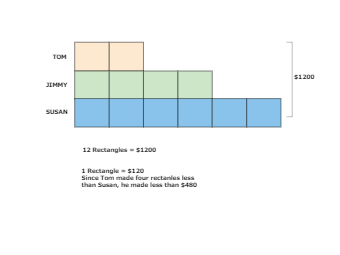• Recent Releases
• Most Viewed
• Most LikedA tape diagram is a diagram commonly used to solve comparison mathematical problems.
Tape diagram math example
7
0
0easy diagrams